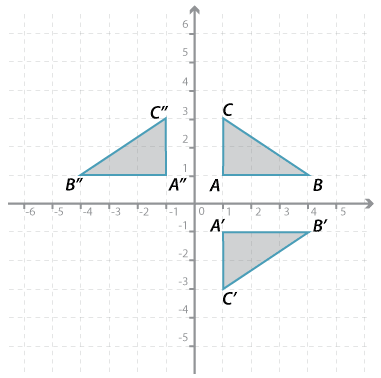## Content description

Describe translations, reflections in an axis, and rotations of multiples of 90 ° on the Cartesian plane using coordinates. Identify line and rotational symmetries (ACMMG181)

Source: Australian Curriculum, Assessment and Reporting Authority (ACARA)

### Reflections using coordinatesDetailed description

A reflection is a transformation that 'flips' a figure about a line. This line is called the axis of reflection.

In the figure above, $$\triangle ABC$$ is reflected in the $$x$$-axis to the image $$\triangle A^\prime B^\prime C^\prime$$. The axis of reflection is the $$x$$-axis.

In the same figure, $$\triangle ABC$$ is reflected in the $$y$$-axis to the image $$\triangle A^{\prime\prime} B^{\prime\prime} C^{\prime\prime}$$. The axis of reflection is the $$y$$-axis.